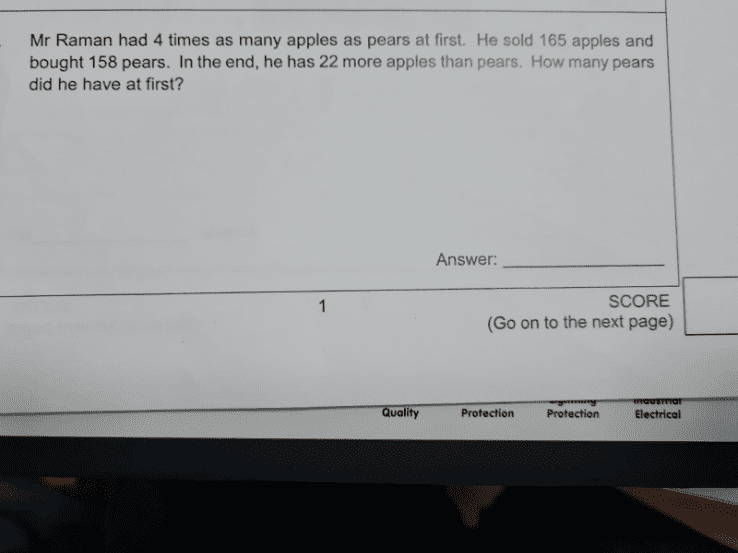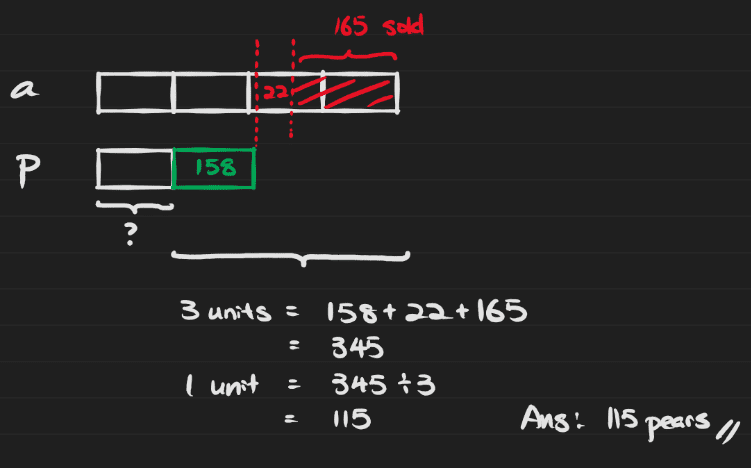# QuestionDear All,

Anyone can help to solve the above question?

Let A be the original number of Apples
Let P be the original number of Pears

(1) A = 4P

Now, (A – 165) – (P + 158) = 22
A – P – 323 = 22
(2) A – P = 345

Substituting (1) into (2):
4P – P = 345
3P = 345
So P = 115 #

I can only do simultaneous equations.  I’d let someone else do the model answer.